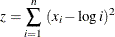### Indexing

Many models have large numbers of variables or parameters that can be categorized into families of similar purpose or behavior. Such families of items can be compactly represented in PROC OPTMODEL by using indexing. You can use indexing to assign each item in such families to a separate value location.

PROC OPTMODEL indexing is similar to array indexing in the DATA step, but it is more flexible. Index values can be numbers or strings, and are not required to fit into some rigid sequence. PROC OPTMODEL indexing is based on index sets, described further in the section Index Sets. For example, the following statement declares an indexed parameter:

   number p{1..3};


The construct that follows the parameter name p, {1..3}, is a simple index set that uses a range expression (see Range Expression). The index set contains the numeric members 1, 2, and 3. The parameter has distinct value locations for each of the index set members. The first such location is referenced as p, the second as p, and the third as p.

The following statements show an example of indexing:

proc optmodel;
number p{1..3};
p=5;
p=7;
p=9;
put p[*]=;


The preceding statements produce a line such as the one shown in Figure 5.5 in the log.

Figure 5.5: Indexed Parameter Output

 p=5 p=7 p=9 

Index sets can also specify local dummy parameters. A dummy parameter can be used as an operand in the expressions that are controlled by the index set. For example, the assignment statements in the preceding statements could be replaced by an initialization in the parameter declaration, as follows:

   number p{i in 1..3} init 3 + 2*i;


The initialization value of the parameter location p is evaluated with the value of the local dummy parameter i equal to 1. So the initialization expression 3 + 2*i evaluates to 5. Similarly for location p, the value of i is 2 and the initialization expression evaluates to 7.

The OPTMODEL modeling language supports aggregation operators that combine values of an expression where a local dummy parameter (or parameters) ranges over the members of a set. For example, the SUM aggregation operator combines expression values by adding them together. The following statements output 21, since p + p + p = 5 + 7 + 9 = 21:

proc optmodel;
number p{i in 1..3} init 3 + 2*i;
put (sum{i in 1..3} p[i]);


Aggregation operators like SUM are especially useful in objective expressions because they can combine a large number of similar expressions into a compact representation. As an example, the following statements define a trivial least squares problem:

proc optmodel;
number n init 100000;
var x{1..n};
min z = sum{i in 1..n}(x[i] - log(i))**2;
solve;


The objective function in this case isEffectively, the objective expression expands to the following large expression:

   min z = (x - log(1))**2
+ (x - log(2))**2
. . .
+ (x - log(99999))**2
+ (x - log(100000))**2;


Even though the problem has 100,000 variables, the aggregation operator SUM enables a compact objective expression.

Note: PROC OPTMODEL classifies as mathematically impure any function that returns a different value each time it is called. The RAND function, for example, falls into this category. PROC OPTMODEL disallows impure functions inside array index sets, objectives, and constraint expressions.